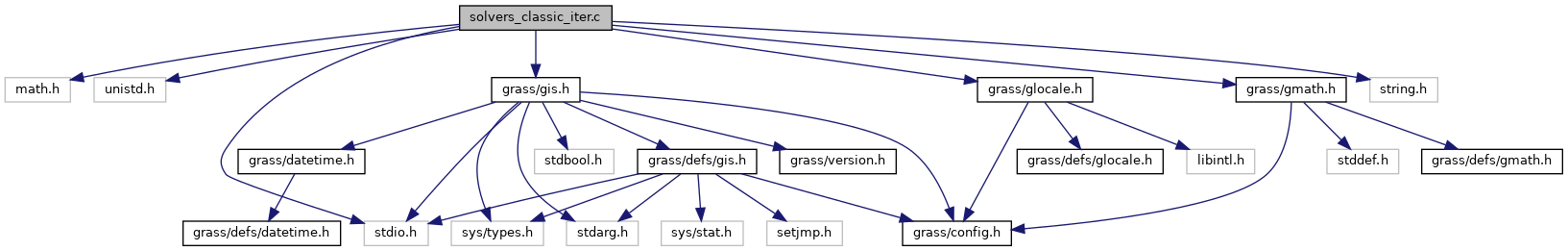GRASS GIS 7 Programmer's Manual  7.9.dev(2021)-e5379bbd7
solvers_classic_iter.c File Reference
`#include <math.h>`
`#include <unistd.h>`
`#include <stdio.h>`
`#include <string.h>`
`#include <grass/gis.h>`
`#include <grass/glocale.h>`
`#include <grass/gmath.h>`
Include dependency graph for solvers_classic_iter.c:Go to the source code of this file.

## Functions

int G_math_solver_sparse_jacobi (G_math_spvector **Asp, double *x, double *b, int rows, int maxit, double sor, double error)
The iterative jacobi solver for sparse matrices. More...

int G_math_solver_sparse_gs (G_math_spvector **Asp, double *x, double *b, int rows, int maxit, double sor, double error)
The iterative gauss seidel solver for sparse matrices. More...

int G_math_solver_jacobi (double **A, double *x, double *b, int rows, int maxit, double sor, double error)
The iterative jacobi solver for quadratic matrices. More...

int G_math_solver_gs (double **A, double *x, double *b, int rows, int maxit, double sor, double error)
The iterative gauss seidel solver for quadratic matrices. More...

## ◆ G_math_solver_gs()

 int G_math_solver_gs ( double ** A, double * x, double * b, int rows, int maxit, double sor, double error )

The iterative gauss seidel solver for quadratic matrices.

The Jacobi solver solves the linear equation system Ax = b The result is written to the vector x.

The parameter maxit specifies the maximum number of iterations. If the maximum is reached, the solver will abort the calculation and writes the current result into the vector x. The parameter err defines the error break criteria for the solver.

Parameters
 A double ** – the dense matrix x double * – the vector of unknowns b double * – the right side vector rows int – number of rows maxit int – the maximum number of iterations sor double – defines the successive overrelaxion parameter [0:2] error double – defines the error break criteria
Returns
int – 1=success, -1=could not solve the les

Definition at line 247 of file solvers_classic_iter.c.

## ◆ G_math_solver_jacobi()

 int G_math_solver_jacobi ( double ** A, double * x, double * b, int rows, int maxit, double sor, double error )

The iterative jacobi solver for quadratic matrices.

The Jacobi solver solves the linear equation system Ax = b The result is written to the vector x.

The parameter maxit specifies the maximum number of iterations. If the maximum is reached, the solver will abort the calculation and writes the current result into the vector x. The parameter err defines the error break criteria for the solver.

Parameters
 A double ** – the dense matrix x double * – the vector of unknowns b double * – the right side vector rows int – number of rows maxit int – the maximum number of iterations sor double – defines the successive overrelaxion parameter [0:1] error double – defines the error break criteria
Returns
int – 1=success, -1=could not solve the les

Definition at line 190 of file solvers_classic_iter.c.

## ◆ G_math_solver_sparse_gs()

 int G_math_solver_sparse_gs ( G_math_spvector ** Asp, double * x, double * b, int rows, int maxit, double sor, double error )

The iterative gauss seidel solver for sparse matrices.

The Jacobi solver solves the linear equation system Ax = b The result is written to the vector x.

The parameter maxit specifies the maximum number of iterations. If the maximum is reached, the solver will abort the calculation and writes the current result into the vector x. The parameter err defines the error break criteria for the solver.

Parameters
 Asp G_math_spvector ** – the sparse matrix x double * – the vector of unknowns b double * – the right side vector rows int – number of rows maxit int – the maximum number of iterations sor double – defines the successive overrelaxion parameter [0:2] error double – defines the error break criteria
Returns
int – 1=success, -1=could not solve the les

Definition at line 118 of file solvers_classic_iter.c.

## ◆ G_math_solver_sparse_jacobi()

 int G_math_solver_sparse_jacobi ( G_math_spvector ** Asp, double * x, double * b, int rows, int maxit, double sor, double error )

The iterative jacobi solver for sparse matrices.

The Jacobi solver solves the linear equation system Ax = b The result is written to the vector x.

The parameter maxit specifies the maximum number of iterations. If the maximum is reached, the solver will abort the calculation and writes the current result into the vector x. The parameter err defines the error break criteria for the solver.

Parameters
 Asp G_math_spvector ** – the sparse matrix x double * – the vector of unknowns b double * – the right side vector rows int – number of rows maxit int – the maximum number of iterations sor double – defines the successive overrelaxion parameter [0:1] error double – defines the error break criteria
Returns
int – 1=success, -1=could not solve the les

Definition at line 48 of file solvers_classic_iter.c.